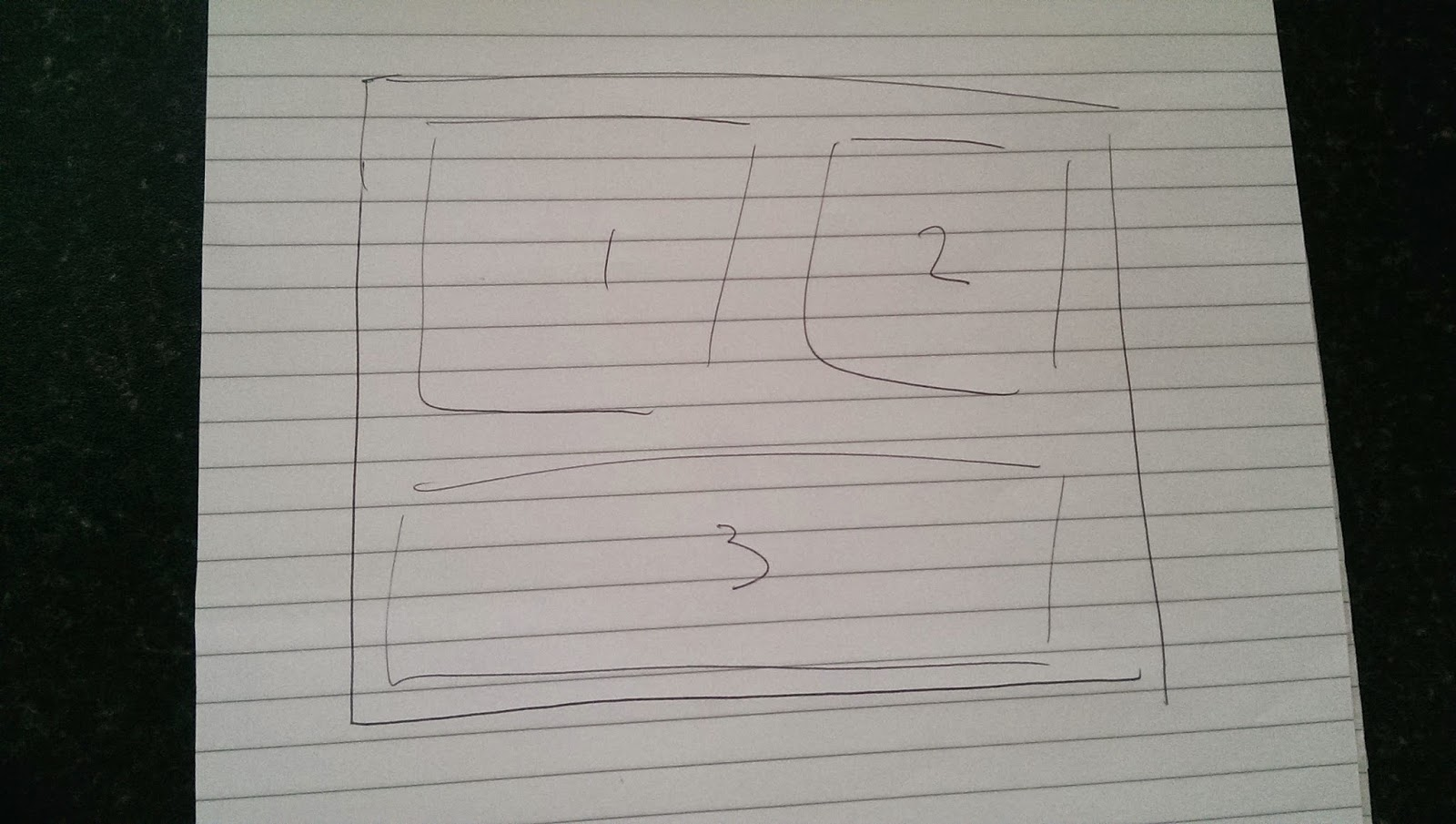## Posts

Showing posts from May, 2014

### Arranging Multiple Plots in RArranging several plots in R as part of a grid isn't always straightforward. Imagine you had three plots and wanted one to stretch along the bottom row and place the other two above (i.e. Figure 1). Figure 1: Sketch of intended placement Using grid.arrange , it is possible to plot several outputs, but the function will automatically place them into a default grid structure (Figure 2). grid.arrange(p1, p2, p3, p4, p5, p6, p7, p8, p9) Figure 2: Using grid.arrange This may be perfectly adequate if they are to be of equal size. However, this isn't always ideal. For example, when aligning the plots below, the result becomes cluttered (Figure 3). grid.arrange(p3, p4, p5) Figure 3: Work in progress As per my original sketch, I want the top plot (with no legend) to run along the bottom row and the other two plots to be positioned side by side above. The following example shows how this can be accomplished. First, a new layout is created, here a